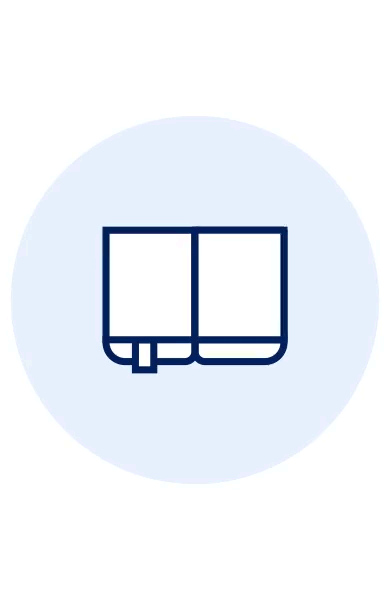MAI SUNT 00:00:00:00

MAI SUNT

X## De (autor): Peter FarrellLearn math by getting creative with code! Use the Python programming language to transform learning high school-level math topics like algebra, geometry, trigonometry, and calculus! Math Adventures with Python will show you how to harness the power of programming to keep math relevant and fun. With the aid of the Python programming language, you'll learn how to visualize solutions to a range of math problems as you use code to explore key mathematical concepts like algebra, trigonometry, matrices, and cellular automata. Once you've learned the programming basics like loops and variables, you'll write your own programs to solve equations quickly, make cool things like an interactive rainbow grid, and automate tedious tasks like factoring numbers and finding square roots. You'll learn how to write functions to draw and manipulate shapes, create oscillating sine waves, and solve equations graphically. You'll also learn how to:
- Draw and transform 2D and 3D graphics with matrices
- Make colorful designs like the Mandelbrot and Julia sets with complex numbers
- Use recursion to create fractals like the Koch snowflake and the Sierpinski triangle
- Generate virtual sheep that graze on grass and multiply autonomously
- Crack secret codes using genetic algorithms As you work through the book's numerous examples and increasingly challenging exercises, you'll code your own solutions, create beautiful visualizations, and see just how much more fun math can be!
Citeste mai mult

-10%

transport gratuit

152.87Lei

169.86 Lei

Sau 15287 de puncteFiecare comanda noua reprezinta o investitie pentru viitoarele tale comenzi. Orice comanda plasata de pe un cont de utilizator primeste in schimb un numar de puncte de fidelitate, In conformitate cu regulile de conversiune stabilite. Punctele acumulate sunt incarcate automat in contul tau si pot fi folosite ulterior, pentru plata urmatoarelor comenzi.

Livrare in 2-4 saptamani

#### Descrierea produsului

Learn math by getting creative with code! Use the Python programming language to transform learning high school-level math topics like algebra, geometry, trigonometry, and calculus! Math Adventures with Python will show you how to harness the power of programming to keep math relevant and fun. With the aid of the Python programming language, you'll learn how to visualize solutions to a range of math problems as you use code to explore key mathematical concepts like algebra, trigonometry, matrices, and cellular automata. Once you've learned the programming basics like loops and variables, you'll write your own programs to solve equations quickly, make cool things like an interactive rainbow grid, and automate tedious tasks like factoring numbers and finding square roots. You'll learn how to write functions to draw and manipulate shapes, create oscillating sine waves, and solve equations graphically. You'll also learn how to:
- Draw and transform 2D and 3D graphics with matrices
- Make colorful designs like the Mandelbrot and Julia sets with complex numbers
- Use recursion to create fractals like the Koch snowflake and the Sierpinski triangle
- Generate virtual sheep that graze on grass and multiply autonomously
- Crack secret codes using genetic algorithms As you work through the book's numerous examples and increasingly challenging exercises, you'll code your own solutions, create beautiful visualizations, and see just how much more fun math can be!
Citeste mai mult

## De pe acelasi raft

Parerea ta e inspiratie pentru comunitatea Libris!

Noi suntem despre carti, si la fel este si